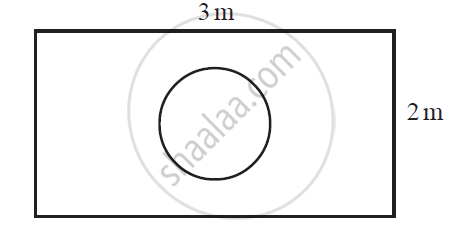Share

# Suppose you drop a die at random on the rectangular region shown in the given figure. What is the probability that it will land inside the circle with diameter 1m? - CBSE Class 10 - Mathematics

ConceptProbability - A Theoretical Approach

#### Question

Suppose you drop a die at random on the rectangular region shown in the given figure. What is the probability that it will land inside the circle with diameter 1m?#### Solution

Area of rectangle = l × = 3 × 2 = 6 m2

Area of circle (of diameter 1 m)  = pir^2= pi((1pi)/2)^2 = pi/4 m^2

P (die will land inside the circle) = (pi/4)/6= pi/24

Is there an error in this question or solution?

#### APPEARS IN

NCERT Solution for Mathematics Textbook for Class 10 (2019 to Current)
Chapter 15: Probability
Ex. 15.10 | Q: 20 | Page no. 310

#### Video TutorialsVIEW ALL 

Solution Suppose you drop a die at random on the rectangular region shown in the given figure. What is the probability that it will land inside the circle with diameter 1m? Concept: Probability - A Theoretical Approach.
S